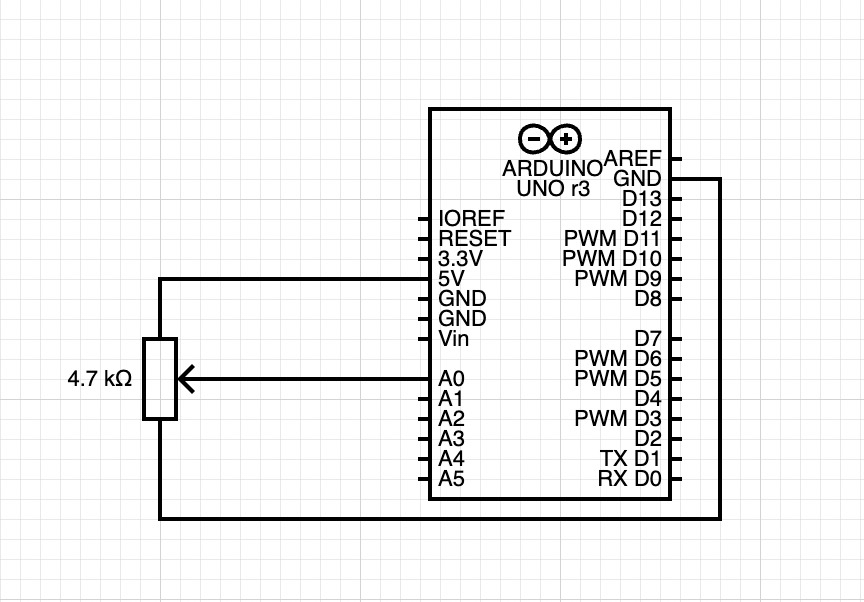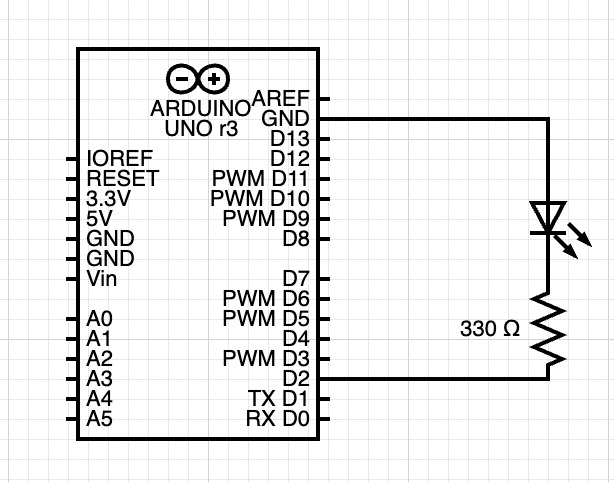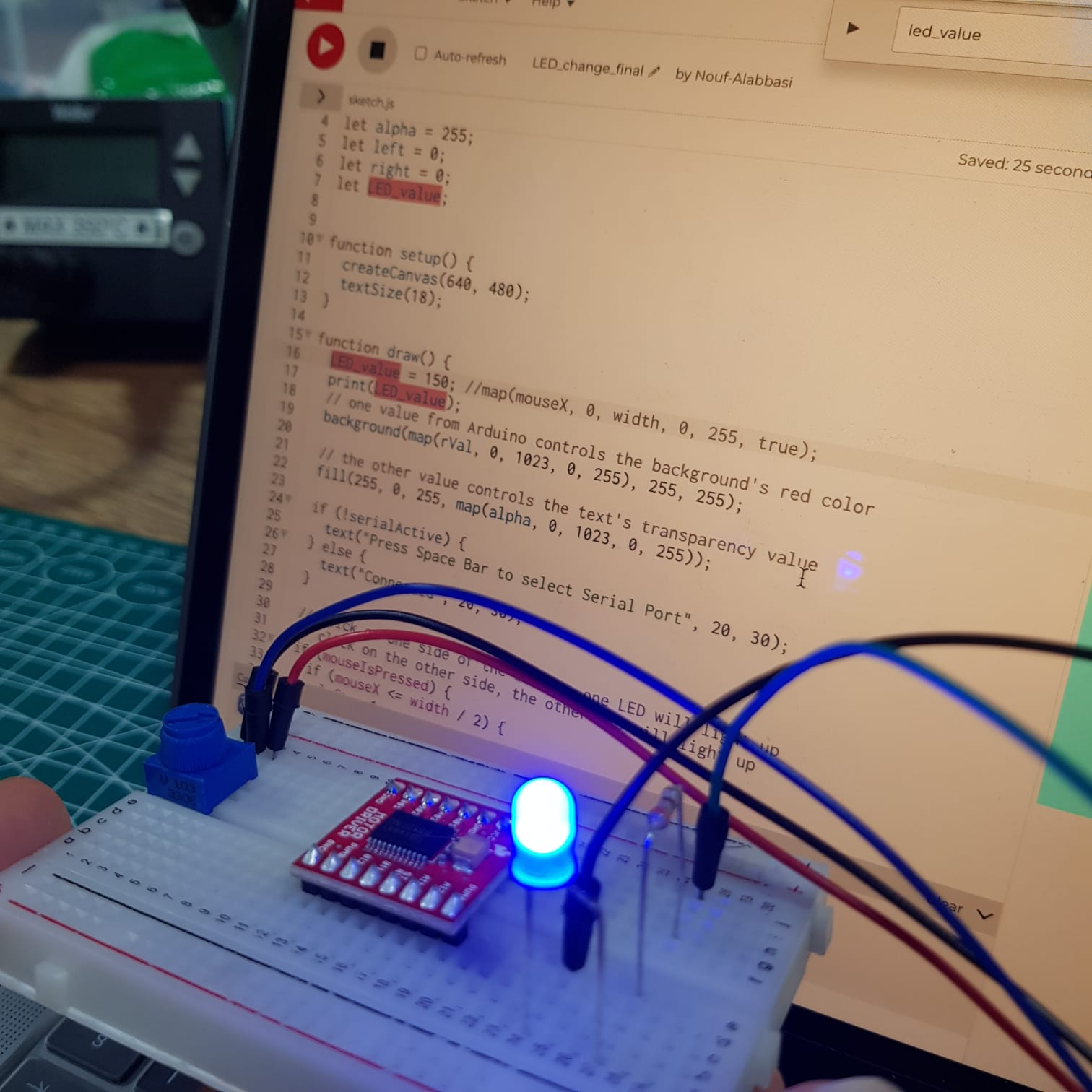# Serial Communication – Nouf and Zhaniya

For the first task, we used potentiometer on our circuit and controlled the X_pos of an ellipse in p5js. We also set up a port choosing thing, so the code requested port connection every time we start the program.
https://editor.p5js.org/Zhaniya/full/ft2iMvilF

Circuit:result:

We used aaron sherwood’s code code which sends and receive data to the arduino. We tried to move those two lines of code

```let sendToArduino = LED_value + "\n";
writeSerial(sendToArduino);```

In the draw function instead of the readSerial() function, but that resulted in a errors with the writeSerial function. We also tried to remove the code in the readSerial function that waits for input from the arduino before sending anything while keeping the two lines of code where it originally is, but that wasn’t working either. We ended up using the original code, but we sent random values to p5js from the arduino and sending the LED brightness value (which is based on where the mouse is horizontally) to the arduino.

```LED_value = map(mouseX, 0, width, 0, 255, true);
print(LED_value);
```

Here is a link to the code: https://editor.p5js.org/Nouf-Alabbasi/sketches/gJtQGnh0w

circuit:the video below shows the LED reacting to the mouse movement (the first video shows the LED and the second Shows the mouse moving)

For the third exercise we followed similar to exercise 2 steps to complete it. We used with gravity wind example with bouncing ball (https://editor.p5js.org/aaronsherwood/sketches/I7iQrNCull) to send the data to the Arduino. After that, we initialized LED_value and set up serial connection.

circuit:##### Problems that we faced:

Some of the problems we faced were simply fixed by uploading the code to the arduino microcontroller but others were a little more challenging to fix. One thing that was not going the way we expected was the LED not gradually changing from one value to another. It seemed to jump from on to off which is not what we were going for.
Here is the p5js code:

```// define the LED brightness as the mouse movement
LED_value = map(mouseX, 0, width, 0, 255, true);
print(LED_value);
…
let sendToArduino = LED_value + "\n";
writeSerial(sendToArduino);
```

And here is the Arduino code:

```…
int LED_val = Serial.parseInt();One hypothesis is perhaps `parseInt` doesn’t handle decimals in numbers, so we expect that it might’ve stopped at the first part of the decimal before the decimal. The numbers should still be usable though, so this doesn’t fully explain why the LED wasn’t being controlled in the way we wanted. (After searching online we found that using `parseFloat` would be a better fit for our code.